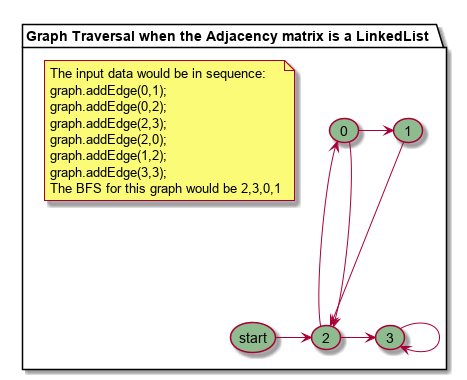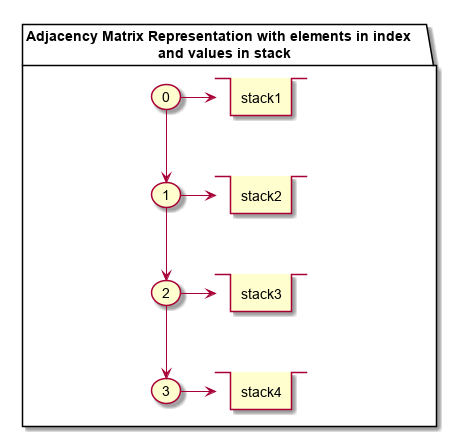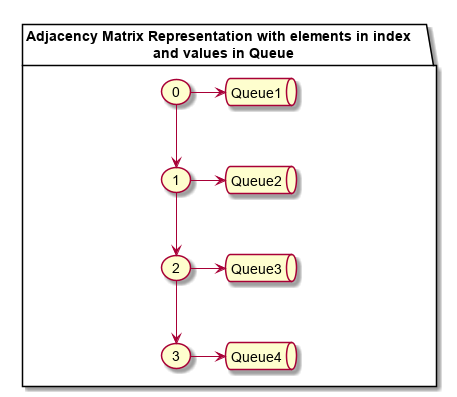{{announcement.body}}
{{announcement.title}}

# Graph BFS With Different DS in Adjacency Matrix and Their Usability

DZone 's Guide to

# Graph BFS With Different DS in Adjacency Matrix and Their Usability

· Java Zone ·
Free Resource

Comment (0)

Save
{{ articles.views | formatCount}} Views

This article analyzes the adjacency matrix used for storing node-link information in an array. For a Graph BFS (Breadth-first-search) traversal, we normally tend to keep an adjacency matrix as a 2D array (`adj[][]`) or array of `linkedLists` as LinkedList[]. As a result, if an element X is added before element Y, it appears in BFS traversal accordingly. BFS traversal becomes like: ~X,Y,~. What if, the appearance of elements within a breath of a graph needs to be traversed  according to some priority or any rule!!

Say in a breath if Priority of Y > Priority of X, element Y should occur before X in BFS traversal. Say In a breath if Y is added later the X, Y should occur before X in traversal.

Let's try to understand with an example the use-cases when we deep dive and tweak this adjacency matrix data structure.

Let's take the below graph as a reference graph, and we would continue to do our analysis with this graph itself.

To understand "Within a breath" in the graph below, then 0,3 are within a breath (same breadth for element 2). This article analyses the appearance of element 0 and element 3's order in BFS traversal. The traversal could be : 2,0,3,1 or 2,3,0,1 depending upon in the insertion of element in graph. What if i wanted a specific order of traversal in-spite the order if insertion.In the graph, since the (2, 3) was added before (2, 0), while traversing BFS, this appears accordingly in a BFS traversal.

BFS Traversal : 2,3,0,1

The Java Code Used for Adjacency matrix with a list:

Java

`xxxxxxxxxx`
1
61

1
`package algorithms.graph.simple.practice;`
2

3
`import java.util.*;`
4

5
`public class GraphDriverWithListMatrix {`
6
`    private static class Graph {`
7
`        private int V;`
8
`        private List<Integer> adj[];`
9
`        Graph(int V) {`
10
`            this.V = V;`
11
`            adj = new LinkedList[V];`
12
`            for(int i = 0; i < V; i++) {`
13
`                adj[i] = new LinkedList<>();`
14
`            }`
15
`        }`
16

17
`        public boolean addEdge(int v, int w) {`
18
`            return adj[v].add(w);`
19
`        }`
20

21
`        public void BFS(int source) {`
22
`            System.out.println(String.format("Following is Breadth First Traversal "+`
23
`                    "(starting from vertex %d)",source));`
24
`        boolean visited[] = new boolean[V];`
25
`        Queue<Integer> queue = new LinkedList<>();`
26

27
`        visited[source] = true;`
28
`        queue.add(source);`
29
`        while(!queue.isEmpty()) {`
30
`            Integer toProcess = queue.poll();`
31
`            System.out.println(toProcess + " ");`
32
`            Iterator<Integer> integerIterator = adj[toProcess].iterator();`
33
`            while(integerIterator.hasNext()) {`
34
`                int n = integerIterator.next();`
35
`                if(!visited[n]) {`
36
`                   visited[n] = true;`
37
`                   queue.add(n);`
38
`                }`
39
`            }`
40
`        }`
41
`        }`
42
`    }`
43

44
`    public static void main(String[] args) {`
45
`        Graph graph = new GraphDriverWithListMatrix.Graph(4);`
46
`        graph.addEdge(0,1);`
47
`        graph.addEdge(0,2);`
48
`        graph.addEdge(2,3);`
49
`        graph.addEdge(2,0);`
50
`        graph.addEdge(1,2);`
51
`        graph.addEdge(3,3);`
52
`        graph.BFS(2);`
53
`    }`
54
`}`
55

56
`Output:`
57
`Following is Breadth First Traversal (starting from vertex 2)`
58
`2 `
59
`3 `
60
`0`
61
`1`

### Use Case 1

Now, let's take a look at a use case where if the X is added before Y, Y should appear before X in BFS traversal. The use case indicates that a stack best suits the requirement.Java

`xxxxxxxxxx`
1
115

1
`package algorithms.graph.simple.practice;`
2

3

4
`import java.util.*;`
5

6
`public class GraphDriverWithStackMatrix {`
7
`    private static class Graph<T extends Indexable> {`
8
`        private int V;`
9
`        private Deque<T> adj[]; /*A Deque is used which is used both as stack and queue. We use it as Stack here.*/`
10
`        Graph(int V) {`
11
`            this.V = V;`
12
`            adj = new ArrayDeque[V];`
13
`            for(int i = 0; i < V; i++) {`
14
`                adj[i] = new ArrayDeque<T>();`
15
`            }`
16
`        }`
17

18
`        public boolean addEdge(int v, T w) {`
19
`            return adj[v].add(w);`
20
`        }`
21

22
`        public void BFS(T source) {`
23
`        System.out.println(String.format("Following is Breadth First Traversal "+`
24
`                    "(starting from vertex %s)",source));`
25
`        boolean visited[] = new boolean[V];`
26
`        Queue<T> queue = new LinkedList<>();`
27
`        visited[source.getIndex()] = true;`
28
`        queue.add(source);`
29
`        while(!queue.isEmpty()) {`
30
`            T toProcess = queue.poll();`
31
`            System.out.println(toProcess + " ");`
32

33
`            Deque<T>[] adjCloned = adj.clone();`
34
`            while(adjCloned[toProcess.getIndex()].peekLast() != null) {`
35
`                T item = adjCloned[toProcess.getIndex()].pollLast();`
36
`                if(visited[item.getIndex()] == false) {`
37
`                    visited[item.getIndex()] = true;`
38
`                    queue.add(item);`
39
`                }`
40
`            }`
41
`        }`
42
`        }`
43
`    }`
44

45
`    public static void main(String[] args) {`
46
`        Graph<Data> graph = new GraphDriverWithStackMatrix.Graph(4);`
47
`        graph.addEdge(0,new Data(1));`
48
`        graph.addEdge(0,new Data(2));`
49
`        graph.addEdge(2,new Data(3));`
50
`        graph.addEdge(2,new Data(0));`
51
`        graph.addEdge(1,new Data(2));`
52
`        graph.addEdge(3,new Data(3));`
53
`       `
54
`        graph.BFS(new Data(2));`
55
`    }`
56

57

58
`}`
59

60
`package algorithms.graph.simple.practice;`
61

62
`import java.util.Optional;`
63

64

65
`class Data implements Indexable{`
66
`    private Integer data;`
67
`    Optional<Integer> priority;`
68
`    public Data(int data){this.data = data; priority = Optional.empty();}`
69
`    public Data(int data, Optional<Integer> priority) {`
70
`        this.data = data;`
71
`        this.priority = priority;`
72
`    }`
73

74
`    public Integer getData() {`
75
`        return data;`
76
`    }`
77

78
`    public void setData(Integer data) {`
79
`        this.data = data;`
80
`    }`
81

82
`    public Optional<Integer> getPriority() {`
83
`        return priority;`
84
`    }`
85

86
`    public void setPriority(Optional<Integer> priority) {`
87
`        this.priority = priority;`
88
`    }`
89

90
`    @Override`
91
`    public int getIndex() {`
92
`        return data;`
93
`    }`
94

95
`    @Override`
96
`    public String toString() {`
97
`        return "Data{" +`
98
`                "data=" + data +`
99
`                '}';`
100
`    }`
101

102
`}`
103

104
`package algorithms.graph.simple.practice;`
105

106
`interface Indexable {`
107
`    int getIndex();`
108
`}`
109

110
`OUTPUT:`
111
`Following is Breadth First Traversal (starting from vertex Data{data=2})`
112
`Data{data=2} `
113
`Data{data=0} `
114
`Data{data=3} `
115
`Data{data=1} `

### Use Case 2

Now, the element has priority associated within a breath. The adjacency matric, in this case, hints about a priority Queue here.Java

x
64

1
`package algorithms.graph.simple.practice;`
2

3

4
`import java.util.*;`
5
`import java.util.concurrent.PriorityBlockingQueue;`
6

7
`public class GraphDriverWithPriorityQueue {`
8
`    private static class Graph<T extends Indexable> {`
9
`        private int V;`
10
`        private PriorityBlockingQueue<T> adj[];`
11
`        Graph(int V) {`
12
`            this.V = V;`
13
`            adj = new PriorityBlockingQueue[V];`
14
`            for(int i = 0; i < V; i++) {`
15
`                adj[i] = new PriorityBlockingQueue<T>(10, (Comparator<? super T>) new DataComparator());`
16
`            }`
17
`        }`
18

19
`        public boolean addEdge(int v, T w) {`
20
`            return adj[v].add(w);`
21
`        }`
22

23
`        public void BFS(T source) {`
24
`        boolean visited[] = new boolean[V];`
25
`        Queue<T> queue = new LinkedList<>();`
26
`        visited[source.getIndex()] = true;`
27
`        queue.add(source);`
28
`        while(!queue.isEmpty()) {`
29
`            T toProcess = queue.poll();`
30
`            System.out.println(toProcess + " ");`
31
`            PriorityBlockingQueue<T>[] adjCloned = adj.clone();`
32
`            while(!adjCloned[toProcess.getIndex()].isEmpty()) {`
33
`                T item = adjCloned[toProcess.getIndex()].poll();`
34
`                if(visited[item.getIndex()] == false) {`
35
`                    visited[item.getIndex()] = true;`
36
`                    queue.add(item);`
37
`                }`
38
`            }`
39
`        }`
40
`        }`
41
`    }`
42

43
`    public static void main(String[] args) {`
44
`        Graph<Data> graph = new Graph(4);`
45
`        graph.addEdge(0,new Data(1));`
46
`        graph.addEdge(0,new Data(2));`
47
`        graph.addEdge(2,new Data(3,Optional.of(2))); /*Priority assigned in the 2nd parameter*/`
48
`        graph.addEdge(2,new Data(0,Optional.of(3)));`
49
`        graph.addEdge(1,new Data(2));`
50
`        graph.addEdge(3,new Data(3));`
51
`        System.out.println("Following is Breadth First Traversal "+`
52
`                "(starting from vertex 2)");`
53
`        graph.BFS(new Data(2));`
54
`    }`
55

56

57
`}`
58
`OUTPUT:`
59
`Following is Breadth First Traversal (starting from vertex Data{data=2})`
60
`Data{data=2} `
61
`Data{data=3} `
62
`Data{data=0} `
63
`Data{data=1} `
64

With Tweaking in Adjacency matrix data-structure, we bring out changes in graph traversal. We shall explore more on tweaking DS and find changes in algorithms in my next algo blog.

Topics:
algorithms ,bfs ,data structures ,graph algorithms ,java

Comment (0)

Save
{{ articles.views | formatCount}} Views

Opinions expressed by DZone contributors are their own.